# Loops

17 de Feb de 2022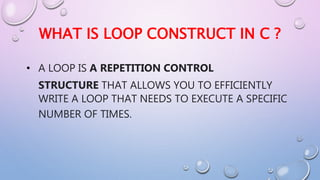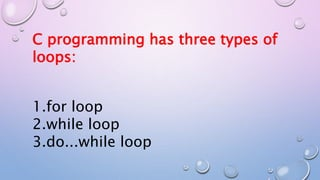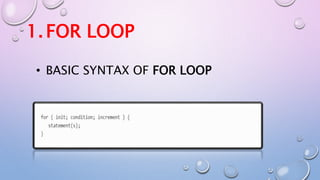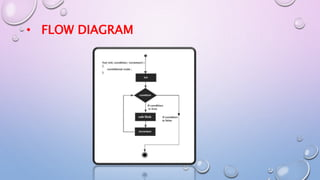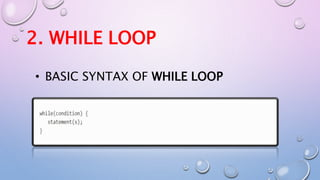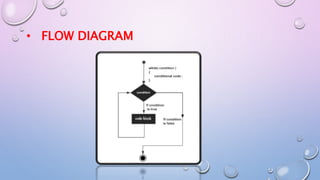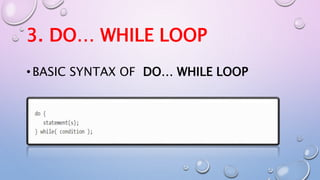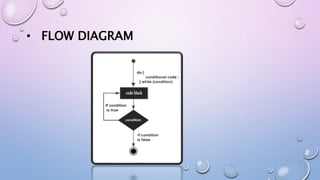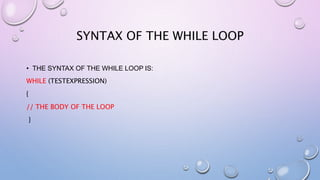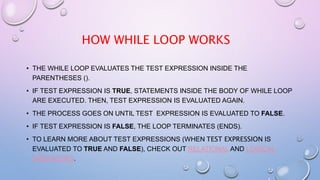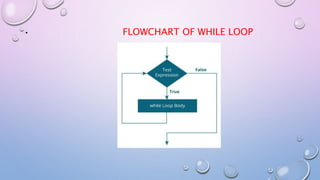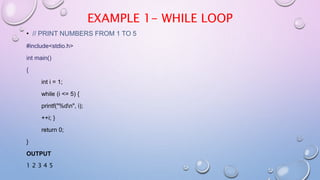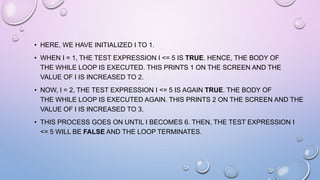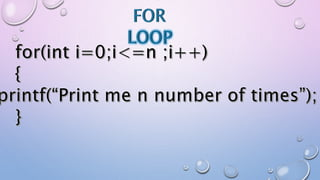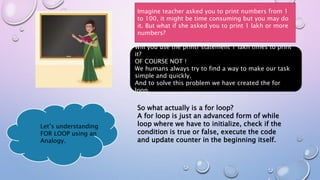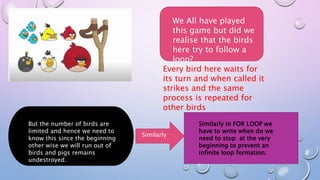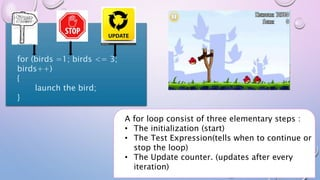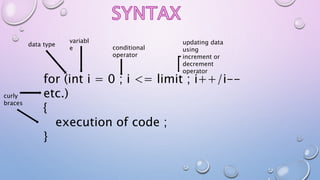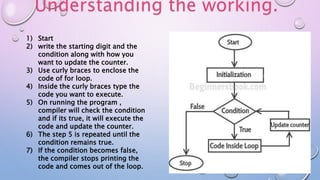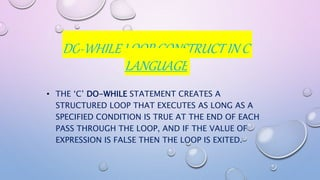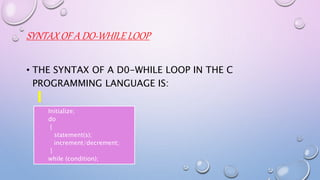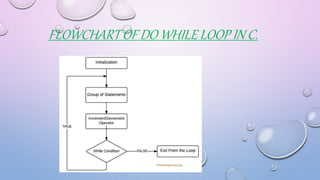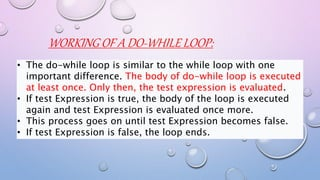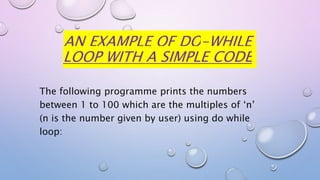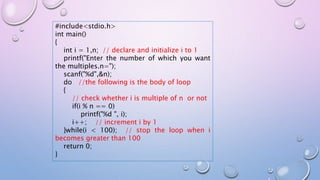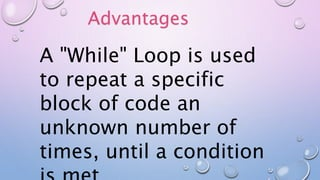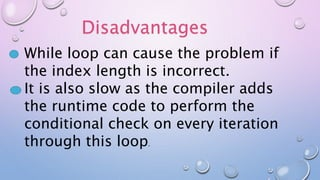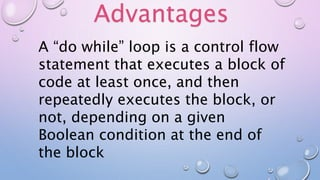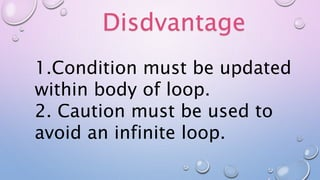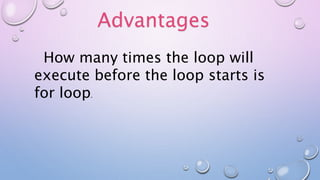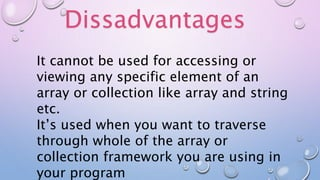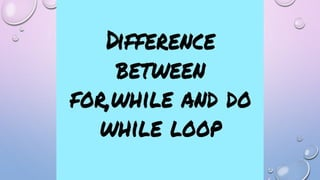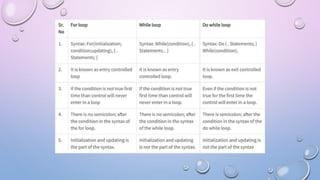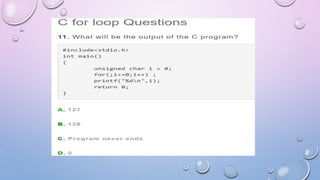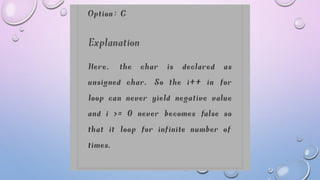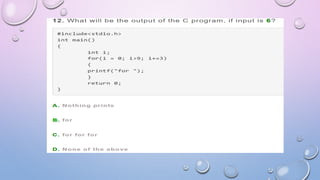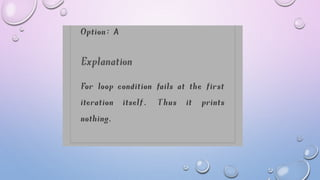1 de 50

### Loops

• 2. WHAT IS LOOP CONSTRUCT IN C ? • A LOOP IS A REPETITION CONTROL STRUCTURE THAT ALLOWS YOU TO EFFICIENTLY WRITE A LOOP THAT NEEDS TO EXECUTE A SPECIFIC NUMBER OF TIMES.
• 3. C programming has three types of loops: 1.for loop 2.while loop 3.do...while loop
• 4. 1.FOR LOOP • BASIC SYNTAX OF FOR LOOP
• 6. 2. WHILE LOOP • BASIC SYNTAX OF WHILE LOOP
• 8. 3. DO… WHILE LOOP •BASIC SYNTAX OF DO… WHILE LOOP
• 10. WHILE LOOP CONSTRUCT IN C A WHILE LOOP IN C PROGRAMMING REPEATEDLY EXECUTES A TARGET STATEMENT AS LONG AS A GIVEN CONDITION IS TRUE
• 11. • THE SYNTAX OF THE WHILE LOOP IS: WHILE (TESTEXPRESSION) { // THE BODY OF THE LOOP } SYNTAX OF THE WHILE LOOP
• 12. HOW WHILE LOOP WORKS • THE WHILE LOOP EVALUATES THE TEST EXPRESSION INSIDE THE PARENTHESES (). • IF TEST EXPRESSION IS TRUE, STATEMENTS INSIDE THE BODY OF WHILE LOOP ARE EXECUTED. THEN, TEST EXPRESSION IS EVALUATED AGAIN. • THE PROCESS GOES ON UNTIL TEST EXPRESSION IS EVALUATED TO FALSE. • IF TEST EXPRESSION IS FALSE, THE LOOP TERMINATES (ENDS). • TO LEARN MORE ABOUT TEST EXPRESSIONS (WHEN TEST EXPRESSION IS EVALUATED TO TRUE AND FALSE), CHECK OUT RELATIONAL AND LOGICAL OPERATORS.
• 13. • FLOWCHART OF WHILE LOOP
• 14. EXAMPLE 1- WHILE LOOP • // PRINT NUMBERS FROM 1 TO 5 #include<stdio.h> int main() { int i = 1; while (i <= 5) { printf("%dn", i); ++i; } return 0; } OUTPUT 1 2 3 4 5
• 15. • HERE, WE HAVE INITIALIZED I TO 1. • WHEN I = 1, THE TEST EXPRESSION I <= 5 IS TRUE. HENCE, THE BODY OF THE WHILE LOOP IS EXECUTED. THIS PRINTS 1 ON THE SCREEN AND THE VALUE OF I IS INCREASED TO 2. • NOW, I = 2, THE TEST EXPRESSION I <= 5 IS AGAIN TRUE. THE BODY OF THE WHILE LOOP IS EXECUTED AGAIN. THIS PRINTS 2 ON THE SCREEN AND THE VALUE OF I IS INCREASED TO 3. • THIS PROCESS GOES ON UNTIL I BECOMES 6. THEN, THE TEST EXPRESSION I <= 5 WILL BE FALSE AND THE LOOP TERMINATES.
• 17. Imagine teacher asked you to print numbers from 1 to 100, it might be time consuming but you may do it. But what if she asked you to print 1 lakh or more numbers? Will you use the printf statement 1 lakh times to print it? OF COURSE NOT ! We humans always try to find a way to make our task simple and quickly. And to solve this problem we have created the for loop. So what actually is a for loop? A for loop is just an advanced form of while loop where we have to initialize, check if the condition is true or false, execute the code and update counter in the beginning itself. Let’s understanding FOR LOOP using an Analogy.
• 18. We All have played this game but did we realise that the birds here try to follow a loop? Every bird here waits for its turn and when called it strikes and the same process is repeated for other birds But the number of birds are limited and hence we need to know this since the beginning other wise we will run out of birds and pigs remains undestroyed. Similarly Similarly in FOR LOOP we have to write when do we need to stop at the very beginning to prevent an infinite loop formation.
• 19. for (birds =1; birds <= 3; birds++) { launch the bird; } A for loop consist of three elementary steps : • The initialization (start) • The Test Expression(tells when to continue or stop the loop) • The Update counter. (updates after every iteration)
• 20. for (int i = 0 ; i <= limit ; i++/i-- etc.) { execution of code ; } data type variabl e conditional operator updating data using increment or decrement operator curly braces
• 21. 1) Start 2) write the starting digit and the condition along with how you want to update the counter. 3) Use curly braces to enclose the code of for loop. 4) Inside the curly braces type the code you want to execute. 5) On running the program , compiler will check the condition and if its true, it will execute the code and update the counter. 6) The step 5 is repeated until the condition remains true. 7) If the condition becomes false, the compiler stops printing the code and comes out of the loop. Understanding the working.
• 22. DO-WHILE LOOP CONSTRUCT IN C LANGUAGE • THE ‘C’ DO-WHILE STATEMENT CREATES A STRUCTURED LOOP THAT EXECUTES AS LONG AS A SPECIFIED CONDITION IS TRUE AT THE END OF EACH PASS THROUGH THE LOOP, AND IF THE VALUE OF EXPRESSION IS FALSE THEN THE LOOP IS EXITED.
• 23. SYNTAX OF A DO-WHILE LOOP • THE SYNTAX OF A D0-WHILE LOOP IN THE C PROGRAMMING LANGUAGE IS: Initialize; do { statement(s); increment/decrement; } while (condition);
• 24. FLOWCHART OF DO WHILE LOOP IN C.
• 25. WORKING OF A DO-WHILE LOOP: • The do-while loop is similar to the while loop with one important difference. The body of do-while loop is executed at least once. Only then, the test expression is evaluated. • If test Expression is true, the body of the loop is executed again and test Expression is evaluated once more. • This process goes on until test Expression becomes false. • If test Expression is false, the loop ends.
• 26. AN EXAMPLE OF DO-WHILE LOOP WITH A SIMPLE CODE The following programme prints the numbers between 1 to 100 which are the multiples of ‘n’ (n is the number given by user) using do while loop:
• 27. #include<stdio.h> int main() { int i = 1,n; // declare and initialize i to 1 printf("Enter the number of which you want the multiples.n="); scanf("%d",&n); do //the following is the body of loop { // check whether i is multiple of n or not if(i % n == 0) printf("%d ", i); i++; // increment i by 1 }while(i < 100); // stop the loop when i becomes greater than 100 return 0; }
• 35. A "While" Loop is used to repeat a specific block of code an unknown number of times, until a condition Advantages
• 36. Disadvantages While loop can cause the problem if the index length is incorrect. It is also slow as the compiler adds the runtime code to perform the conditional check on every iteration through this loop.
• 38. A “do while” loop is a control flow statement that executes a block of code at least once, and then repeatedly executes the block, or not, depending on a given Boolean condition at the end of the block Advantages
• 39. Disdvantage 1.Condition must be updated within body of loop. 2. Caution must be used to avoid an infinite loop.
• 41. Advantages How many times the loop will execute before the loop starts is for loop.
• 42. Dissadvantages It cannot be used for accessing or viewing any specific element of an array or collection like array and string etc. It’s used when you want to traverse through whole of the array or collection framework you are using in your program